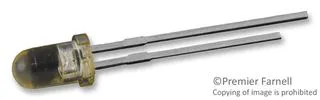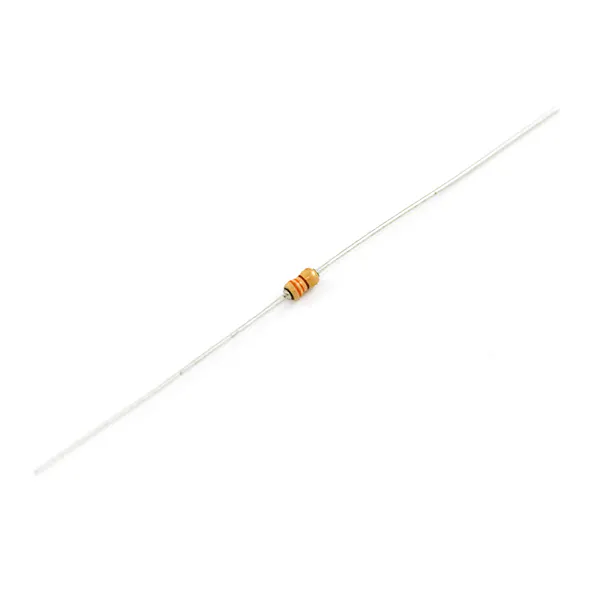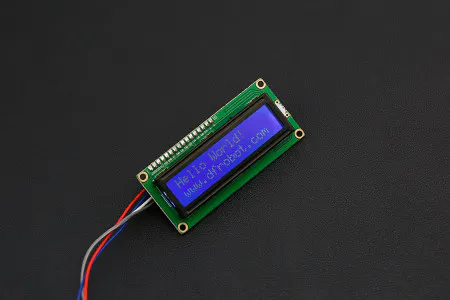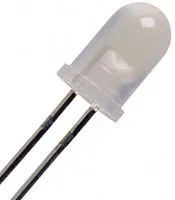Project tutorial

# Really Homemade Oximeter Sensor © CC BY-NC

How to make an oximeter sensor to measure heartbeat and oxygen saturation in blood, using a few components that any maker already has.

• 70,468 views
• 59 respects

## Components and suppliesInfrared Emitter, 18 ° Wavelength between 805nm and 1000nm
×1
 Photodiode LPT80A The photodiode needed is a wide range one, it must be able to receive both red signals (600nm) and infrared signals (940nm).
×15 mm LED: Red Wavelength near 600nm
×1Resistor 10k ohm
×1Resistor 330 ohm
×2DFRobot I2C 16x2 Arduino LCD Display Module Or another lcd display to show values.
×1Arduino UNO
×1Jumper wires (generic)
×1High Brightness LED, White Optional third led, see improvement section of the project
×1

## Apps and online services

In this period of isolation, I've built an oximeter with parts already in house. An oximeter after all is just made by two leds and a photodiode.

I'm not an expert of medical knowledge and at this stage of the project I'm notsure that this work has a diagnostic value, but it's a good educational project to study how it works, and probably with a few tips it could become an homemade medical tool.

### Oxygen saturation and COVID-19

In this incredible period of our life we've learned a lot of things about viruses, lungs, surgical masks, soap and washing hands. Everybody reads about symptoms like coughing, fever and breathing difficulties. We've also known that one way to measure breathing difficulty is reading the amount of oxygen in our blood.

This measure can be read indirectly with a medical device called Oximeter. You have probably already seen it, it's a non invasive device that is placed on a finger with some pulsating lights that do the work. Like this:

Normally, when you're ok, you have a percentage of oxygen saturation (SpO2) near or grater than 95%. When saturation goes down below 90% and you have cough and fever, it's a problem.

If any maker could build an oximeter, discover an infection would be easier and could help people to decide to go to hospital when the problem really exists and not for a panic attack.

### First, understand how heartbeat sensor works

I've started this project playing with a KY-039 heartbeat sensor that I've found in a kit sensor that many of us have in home. As you can see in the circuit below, it's just a infrared led that lights a photodiode. There are also two resistors to protect the led and read the small signal of the sensor.

So if you don't have a KY-039 sensor you can build your own sensor with few components.

The finger is placed between the sensor and the photodiode like in this photo (originally taken from this site and modified):

The light emitted by the infrared led is partially absorbed by the nail, the skin, and all the other parts of your finger, but it's not constant because it changes following the changes of the blood running in your veins. When your heart makes a beat the blood is pushed in your veins and the light absorption changes. We can measure the current generated by the photodiode illuminated by the infrared light that reach it.

The KY-039 sensor has a S (signal) pin to read that changing value.

### We can measure heartbeat rate by counting peaks of the signal

Reading a value from a variable signal from a sensor it's not so easy, because there is a lot of noise, the signal is really low, and we need to make some math to find the good values to plot.

I've got to thank this useful post from Johan Ha, which explains how to calculate the average of the signal and it also explains how to remove the noise made by a home lamp (that light is a noise!).

The trick is to make an array in which we push a value and drop a value to make the average of the last X values read from the sensor. He has also describes a way to find the rising of the signal, by counting N growing values. I mean, when a value is grater than the preceding value for N times, it's a peak.

Using the Arduino Serial plot tool or another serial tool to analyze values printed on COM port (such as SerialPlot), and trying different values we can define a correct number N (`rise_threshold` constant n the code). If you define a number too big or too small you can miss some beats or count a dicrotic notch as a beat.

Once you've understand how to fine the peaks, just count them, or calculate the time between a small serie of beats to determine your `BPM`rate (Beats Per Minute).

### Building the oximeter (hacking the KY-039 sensor) to find oxygen saturation

Our blood absorbs light in a different way with the change of the wave-length of the light. The red light (`~600nm`) is absorbed better by the blood that contains more oxygen, so we can compare the measures made with infrared led (`~950nm`) with the ones made with red led and find the percentage of oxygen in our blood. That value is called `Sp02%` (peripheral capillary oxygen saturation).

Since I have a KY-039 sensor I've decided to modify it. It has just an infrared led, so I've added a RED led, disconnect the IR led from the Vcc and connect with a `330 ohm` resistor the two leds to two different pins of Arduino.

(If you don't have a KY-039 sensor tomodify you can build it, it's just a couple of leds, a photodiode and 3 resistors, and the schematic is really simple!)

Here is the schematic of the modified sensor:

In this way we can turn on the IR led and read the value from the KY-039 S pin, then we can turn off the IR led and turn on the RED led, and read the value from the KY-039 S pin.

Here it is mine:

If you plot the two signals you can see that IR values are always lower than Red values.

To find a good signal remember to place the fingertip correctly on the photodiode and the leds should touch the nail, when you find a comfortable position with good reading on the plot do not change it.

Since the signals are low and noise is very problematic, to get useful mesures I've noticed that a good ambient light is always needed. So, don't move your finger while measuring and don't change the light, just a shadow on the sensor could change everything.

### How is measured saturation SpO2%

The oxygen saturation level (SpO2) is the fraction of oxygen-saturated hemoglobin relative to total hemoglobin and is a function of a parameter called R (I've found this information in an academic paper from Politecnico of Milan), which is calculated using minimum and maximum values from the two signals:

`R = ( (REDmax-REDmin) / REDmin ) / ((IRmax-IRmin) / IRmin)`

Each instrument has it's own R and it's needed calibration to find the curve (the function) that connects R with SpO2%.

We've counted the number of peaks, but now we need to find max and min values of the two curves (RED led and IR led).

To accomplish this job we evaluate the "period" of the heartbeat (that is to say how many milliseconds a beat lasts) and divide it for the sampling rate to determine how many samples make a period. The sampling rate is in our case 40 milliseconds because we read the IR led for 20 milliseconds and then the RED led of another 20 milliseconds.

The period of the beat is the time that passes between two rising curves in the signal.

So I can analyze the last L samples (where L = period / 40), which I have saved in an array, to find REDmax, REDmin, IRmax and IRmin values.

With maximum and minimum values I can calculate R.

R, L and period are calculated every beat, so the calculus of R is also done for every beat.

### From R to SpO2%: How to calibrate the oximeter?

The function that links R with SpO2 can be simplified with a straight line:

`SpO2 = K * R + M`

So we need two points (two couples of value of SpO2 and R) to determine K and M. The only way to find these 2 points is using another oximeter and read the values from its display.

The new oximeter will be the reference, we read the SpO2 value while measuring the R values from our homemade oximeter.

First breath normally, and read the value of SpO2 and R. Write it down.

Then try to keep the breath and after a 10-20 seconds you will read SpO2 in the new oximeter decreasing, you should also see the R parameter of your oximeter growing. Before faint, write down the values of SpO2 reached and the value of your R parameter.

Solve the 2nd degree equation and found K and M for your oximeter.

Now it's possible to calculate both bpm and SpO2 values for every measure of R.

I've also added a display to show all the the numbers, I show values only if I've found at least 5 measures of periods that doesn't change too much (±`10%` of the period length). In this way I remove values that changes too much that depends on the poor components or change of ambient lights or finger movement.

The c value indicates that the values shown are calculated with c stable measures.

### Project improvement: remove the ambient light variability

After a few days of playing with my project, I've found a way to improve it.

I've notice that with these low costs components (we're using just leds and a photo diode!) the measures are too much ambient-light dependents and this is not a good thing, if we want to read data properly in a real working environment. Since I've notice that in a sunny day the results are better than with cloudy light or in the evening when I use an electrical lamp, I've decided to add a third led, which is always on and provides just light on the finger.

With this 3-leds-sensor the measures are also taken under a black cloth to exclude the ambient light which could always change.

Now, the results are better and do not depend anymore on the ambient light.

I've also had to re-calibrate the oximeter, as you can see from the video after few seconds it correctly finds bpm ans SpO2%:

## Code

##### oximeter-diy-ver-0.92.inoArduino
This is the source code of the Oximeter DIY, which is made with few components that a maker could have in home.
```/*
* an oximeter diy. v.0.92 (minor fixes)
* by hacking a ky-039 heartbeat sensor or using an infrared led
* a red led and a photodiode.
*/

#include <Wire.h>
#include <LiquidCrystal_I2C.h>

#define maxperiod_siz 80 // max number of samples in a period
#define measures 10      // number of periods stored
#define samp_siz 4       // number of samples for average
#define rise_threshold 3 // number of rising measures to determine a peak

// a liquid crystal displays BPM
LiquidCrystal_I2C lcd(0x3F, 16, 2);

int T = 20;              // slot milliseconds to read a value from the sensor
int sensorPin = A1;
int REDLed = 3;
int IRLed = 4;

byte sym = {
{
B00000,
B01010,
B11111,
B11111,
B01110,
B00100,
B00000,
B00000
},{
B00000,
B00000,
B00000,
B11000,
B00100,
B01000,
B10000,
B11100
},{
B00000,
B00100,
B01010,
B00010,
B00100,
B00100,
B00000,
B00100
}
};

void setup() {
Serial.begin(9600);
Serial.flush();
pinMode(sensorPin,INPUT);
pinMode(REDLed,OUTPUT);
pinMode(IRLed,OUTPUT);

// initialize the LCD
lcd.init();
lcd.backlight();

// turn off leds
digitalWrite(REDLed,LOW);
digitalWrite(IRLed,LOW);

for(int i=0;i<8;i++) lcd.createChar(i, sym[i]);

}

void loop ()
{

bool finger_status = true;

int period, samples;
period=0; samples=0;
int samplesCounter = 0;
int ptrMM =0;
for (int i = 0; i < maxperiod_siz; i++) { readsIRMM[i] = 0;readsREDMM[i]=0;}
float IRmax=0;
float IRmin=0;
float REDmax=0;
float REDmin=0;
double R=0;

float measuresR[measures];
int measuresPeriods[measures];
int m = 0;
for (int i = 0; i < measures; i++) { measuresPeriods[i]=0; measuresR[i]=0; }

int ptr;

float beforeIR;

bool rising;
int rise_count;
int n;
long int last_beat;
for (int i = 0; i < samp_siz; i++) { readsIR[i] = 0; readsRED[i]=0; }
sumIR = 0; sumRED=0;
ptr = 0;

while(1)
{

//
// turn on IR LED
digitalWrite(REDLed,LOW);
digitalWrite(IRLed,HIGH);

// calculate an average of the sensor
// during a 20 ms (T) period (this will eliminate
// the 50 Hz noise caused by electric light
n = 0;
start = millis();
do
{
n++;
}
while (millis() < start + T);
reader /= n;  // we got an average
// Add the newest measurement to an array
// and subtract the oldest measurement from the array
// to maintain a sum of last measurements
lastIR = sumIR / samp_siz;

//
// TURN ON RED LED and do the same

digitalWrite(REDLed,HIGH);
digitalWrite(IRLed,LOW);

n = 0;
start = millis();
do
{
n++;
}
while (millis() < start + T);
reader /= n;  // we got an average
// Add the newest measurement to an array
// and subtract the oldest measurement from the array
// to maintain a sum of last measurements
lastRED = sumRED / samp_siz;

//
// R CALCULATION
// save all the samples of a period both for IR and for RED
ptrMM++;
ptrMM %= maxperiod_siz;
samplesCounter++;
//
// if I've saved all the samples of a period, look to find
// max and min values and calculate R parameter
if(samplesCounter>=samples){
samplesCounter =0;
IRmax = 0; IRmin=1023; REDmax = 0; REDmin=1023;
for(int i=0;i<maxperiod_siz;i++) {
}
R =  ( (REDmax-REDmin) / REDmin) / ( (IRmax-IRmin) / IRmin ) ;
}

// check that the finger is placed inside
// the sensor. If the finger is missing
// RED curve is under the IR.
//
if (lastRED < lastIR) {
if(finger_status==true) {
finger_status = false;
lcd.clear();
lcd.setCursor(0,0);
lcd.print("No finger?");
}
} else {
if(finger_status==false) {
lcd.clear();
finger_status = true;

lcd.setCursor(10,0);
lcd.print("c=");

lcd.setCursor(0,0);
lcd.print("bpm");
lcd.setCursor(0,1);
lcd.print("SpO"); lcd.write(1);  //2
lcd.setCursor(10,1);
lcd.print("R=");

}
}

float avR = 0;
int avBPM=0;

if (finger_status==true){

// lastIR holds the average of the values in the array
// check for a rising curve (= a heart beat)
if (lastIR > beforeIR)
{

rise_count++;  // count the number of samples that are rising
if (!rising && rise_count > rise_threshold)
{
lcd.setCursor(3,0);
lcd.write( 0 );       // <3
// Ok, we have detected a rising curve, which implies a heartbeat.
// Record the time since last beat, keep track of the 10 previous
// peaks to get an average value.
// The rising flag prevents us from detecting the same rise
// more than once.
rising = true;

measuresR[m] = R;
measuresPeriods[m] = millis() - last_beat;
last_beat = millis();
int period = 0;
for(int i =0; i<measures; i++) period += measuresPeriods[i];

// calculate average period and number of samples
// to store to find min and max values
period = period / measures;
samples = period / (2*T);

int avPeriod = 0;

int c = 0;
// c stores the number of good measures (not floating more than 10%),
// in the last 10 peaks
for(int i =1; i<measures; i++) {
if ( (measuresPeriods[i] <  measuresPeriods[i-1] * 1.1)  &&
(measuresPeriods[i] >  measuresPeriods[i-1] / 1.1)  ) {

c++;
avPeriod += measuresPeriods[i];
avR += measuresR[i];

}
}

m++;
m %= measures;

lcd.setCursor(12,0);
lcd.print(String(c)+"  ");

// bpm and R shown are calculated as the
// average of at least 5 good peaks
avBPM = 60000 / ( avPeriod / c) ;
avR = avR / c ;

// if there are at last 5 measures
lcd.setCursor(12,1);
if(c==0) lcd.print("    "); else lcd.print(String(avR) + " ");

// if there are at least 5 good measures...
if(c > 4) {

//
// SATURTION IS A FUNCTION OF R (calibration)
// Y = k*x + m
// k and m are calculated with another oximeter
int SpO2 = -19 * R + 112;

lcd.setCursor(4,0);
if(avBPM > 40 && avBPM <220) lcd.print(String(avBPM)+" "); //else lcd.print("---");

lcd.setCursor(4,1);
if(SpO2 > 70 && SpO2 <150) lcd.print( " " + String(SpO2) +"% "); //else lcd.print("--% ");

} else {
if(c <3) {
// if less then 2 measures add ?
lcd.setCursor(3,0); lcd.write( 2 ); //bpm ?
lcd.setCursor(4,1); lcd.write( 2 ); //SpO2 ?
}
}

}
}
else
{
// Ok, the curve is falling
rising = false;
rise_count = 0;
lcd.setCursor(3,0);lcd.print(" ");
}

// to compare it with the new value and find peaks
beforeIR = lastIR;

} // finger is inside

// PLOT everything
Serial.print(lastIR);
Serial.print(",");
Serial.print(lastRED);
/*
* Serial.print(",");
Serial.print(R);
Serial.print(",");
Serial.print(IRmax);
Serial.print(",");
Serial.print(IRmin);
Serial.print(",");
Serial.print(REDmax);
Serial.print(",");
Serial.print(REDmin);
Serial.print(",");
Serial.print(avR);
Serial.print(",");
Serial.print(avBPM); */
Serial.println();

// handle the arrays
ptr++;
ptr %= samp_siz;

} // loop while 1
}
```

## Schematics

In my project I've modified a KY-039 but that sensor isn't available in the fritzing library so I've build it with the few components that is made of but I didn't found a proper photodiode in the fritizing library.
oximeter-diy_oW9ZI5zQtJ.fzz
A simple oximeter to read oxygen in the blood can be made hacking the KY-039 sensor, or by building a sensor from scratch,#### Open Source Pulse Oximeter for COVID-19

Project tutorial by Arduino “having11” Guy

• 81,816 views
• 141 respects

• 13,747 views
• 11 respects

#### How to make a thermometer with Arduino and the LM35 sensor

Project tutorial by Robotics Arduino

• 1,991 views
• 3 respects

#### DHT11 Temperature/Humidity Sensor

Project tutorial by Zachary Haslam

• 173,420 views
• 114 respects

#### How to Make an IR Sensor

Project tutorial by Manikant savadatti

• 26,654 views